# The N-queens Problem¶

Oct. 8, 2018

The N-queens problem is a classic problem that asks the following question.

How can N queens be place on a NxN chessboard so that no two queens attack each other?

The following figure shows a possible solution to the N-queens problem for $N=8$.The N-queens problem can be solve via recursively searching a rooted tree using the depth-first scheme. This is a type of combinatorial optimization problem, where the optimal solution is found by searching through a very large set of possible solutions. Whenever the search yields a solution, or finding that a solution is not possible under current node, the search backtracks to the previous stage and changes state of a variable that hasn't been tried before. In this post, we will implement a simple recursive solution to finding the number of solution to the N-queens problem.

First, we define a function that builds a NxN chessboard. This can be easily achieved as a numpy array.

In :
import numpy as np
import matplotlib.pyplot as plt
import pandas as pd
import seaborn as sns; sns.set()
%matplotlib inline

In :
def build_chessboard(N):
return np.zeros((N, N))


Next, we define a printing function that displays a heatmap of the chessboard.

In :
def print_board(chessboard):
df = pd.DataFrame(chessboard)
plt.figure()
sns.heatmap(df, linewidth=0.5, cbar=False, cmap="viridis",
square=True, xticklabels=False, yticklabels=False)


The following fill_queen function is used to label all cells that are under attack by a queen.

In :
def fill_queen(chessboard, row, col):
N = len(chessboard)
chessboard[:, col] = 2
chessboard[row, :] = 2
for i in range(N):
try:
chessboard[row+i, col+i] = 2
except IndexError:
pass
if (row-i>=0):
try:
chessboard[row-i, col+i] = 2
except IndexError:
pass
if (row-i>=0) and (col-i)>=0:
try:
chessboard[row-i, col-i] = 2
except IndexError:
pass
if (col-i>=0):
try:
chessboard[row+i, col-i] = 2
except IndexError:
pass
chessboard[row,col] = 1
return chessboard


Let's test our functions by building a 9x9 chessboard and placing a queen at the center.

In :
chessboard = build_chessboard(9)
fill_queen(chessboard, 4, 4)
print_board(chessboard)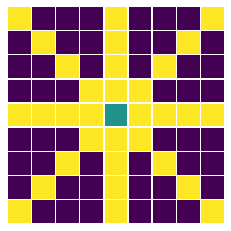Now we are ready to build the recursive function to find the number of solutions.

In :
def N_queens(chessboard, col=0, count=0, solutions=[]):
"""
Inputs:
------------
chessboard: NxN chessboard as numpy array
col: column number from 0 to N (start at 0)
count: total number of solutions (start at 0)

Output:
------------
The number of solutions.
Also plots the first five solutions.
"""

# Building a NxN chessboard
N = len(chessboard)

if col == N:
# Appending a new solution to the list
solutions.append(chessboard)
# Printing the first five solutions
if count < 5:
print_board(chessboard)
return count+1, solutions

# The traversal set of a tree as rows
for row in range(N):
# Check whether a cell is under attack. If not, place a queen.
if chessboard[row][col] == 0:
# Creating a copy of the chessboard so that the original remains in the tree
'''
This is a crucial step. Without copying, the original root
of the tree will be changed, so we lose the tree structure!
'''
chessboard_copy = chessboard.copy()
solutions_copy = solutions.copy()
# Placing a new queen
chessboard_copy = fill_queen(chessboard_copy, row, col)
# Branching to a lower level in the tree
count, solutions = N_queens(chessboard_copy, col+1, count, solutions_copy)

return count, solutions

In :
chessboard = build_chessboard(8)
count, solutions = N_queens(chessboard)
print ("Number of solutions:", count)

Number of solutions: 92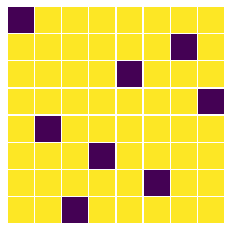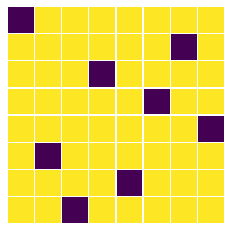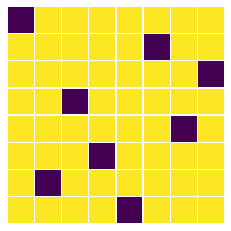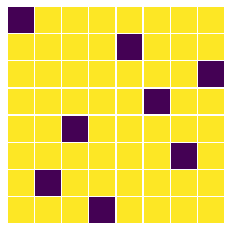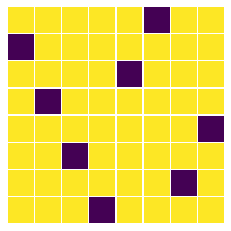To build an animated version of all the solutions, all you need is to install the ImageMagick package. On Ubuntu, you can simply type in the terminal,

sudo apt-get install imagemagick


On Mac OSX, we can use homebrew by typing in the terminal,

brew install imagemagick


Then run the following code, and the output will be a .gif of all the solutions. (Note that this only works on UNIX systems.)

In :
i = 0
for chessboard in solutions:
print_board(chessboard)
plt.savefig(str(i)+'myfig')
i += 1
plt.close()

from subprocess import Popen
Popen('convert -delay 20 -loop 0 *.png myimage.gif', shell=True).wait()
Popen('rm *myfig.png', shell=True).wait()
pass


The output is the following.